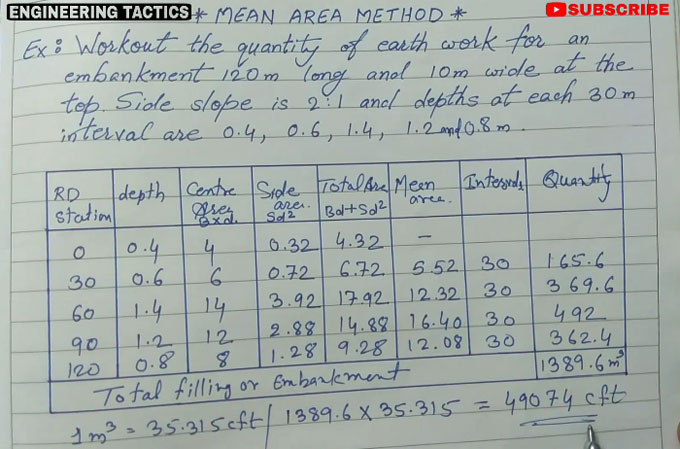# How to calculate quantity of earthwork in roadIn this civil engineering video tutorial, you will learn step-by-step guidelines for measuring the quantity of earthwork (soil) in road, railway, canal work with the help of mean area method.

For explanation, the solution is given on the following example :-

Example – Calculate the quantity of earthwork for an embankment with length 120 m and width 10 m at the top. Side slope is given as 2:1 and depths at each 30 m distance are 0.4, 0.6, 1.4, 1.2 and 0.8 m.

The calculation is done through a table. The table contains various heads like Road station, depth, centre area, side area, total area, mean area, intervals and quantity.

The work of road will start from 0 point. So, in road station column in the table, the values will be given as 0, 30, 60, 90, 120 (as distance is given as 30 m).

In depth column, provide the values as 0.4, 0.6, 1.4, 1.2 and 0.8 m

To calculate center area, the following formula will be used :-
B x d = Breadth or width x Depth

After putting the values, we get the following result :-
10 x 0.4 = 4 meter
In this way, the other values can be determined easily.

To determine the side area, the following formula will be used :-
Sd2, here S denotes slope and d denotes depth.

After putting the values, we get the following output :-
2 x 0.42 = 0.32 m2 (as sloe is given by 2:1)
In this way, the other values can be determined easily.

Total area will be calculated with the following formula :-
Bd + Sd2 = Center Area + Side area
To determine the mean area, first, sum up the first two rows of total area and then divide it with 2 i.e. 4.32 + 6.72 /2 = 5.52 meter. In this way, other values can be calculated.
The value of intervals is given as 30 meter.

Finally, the total area will be calculated as follow :-
Mean area x Intervals

After putting the values, we get the following result :-
5.52 x 30 = 165.6 m2
In this way, the other values can be calculated.
Total filling or embankment will be determined by summing up all the quantities and it will be 1389.6 m3
So, the total quantity of soils for filling or embankment of the road = 1389.6 m3

To get more clear ideas, go through the following video tutorial.

Video Source: NGINEERING TACTICS